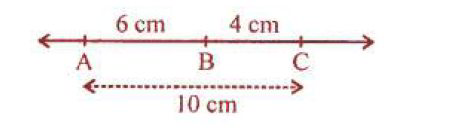# ML Aggarwal Solutions Class 6 Mathematics Solutions for Understanding Symmetrical Shapes Exercise 11 in Chapter 11 - Understanding Symmetrical Shapes

Question 3 Understanding Symmetrical Shapes Exercise 11

If A, B and C are collinear points such that AB = 6 cm, BC = 4 cm and AC = 10 cm,

which one of them lies between the other two?

From the question it is given that,

A, B and C are collinear points

AB = 6 cm

BC = 4 cm

AC = 10 cmRelated Questions

Lido

Courses

Teachers

Book a Demo with us

Syllabus

Maths
CBSE
Maths
ICSE
Science
CBSE

Science
ICSE
English
CBSE
English
ICSE
Coding

Terms & Policies

Selina Question Bank

Maths
Physics
Biology

Allied Question Bank

Chemistry
Connect with us on social media!